• ## C语言注册登录系统

万次阅读 多人点赞 2015-10-22 18:33:05
C语言实现的注册登录系统，系统未注册之前，提示先注册，注册的时候限制用户名10个字符之内，密码为8位，登录系统的时候，三次机会，只有用户名和密码都正确的时候，才能够登录系统
/* Note:Your choice is C IDE */
#include "stdio.h"
#include "string.h"
#include "windows.h"

//定义变量
char reg_name="",reg_pwd="";
char on_name,on_pwd;

//用户注册系统
void regist()
{
//清屏
system("pause");
system("cls");

printf("\n\n\t\t\t欢迎使用腾讯QQ注册系统\n\n");
while(1)
{
//输入用户名
printf("\t\t请输入用户名[不能大于10个字符]：");
scanf("%s",reg_name);

//判断用户名
if(strlen(reg_name)<=10)
{
while(1)
{
//输入密码
printf("\n\t\t请输入密码[密码长度为八位]：");
scanf("%s",reg_pwd);

//判断密码
if(strlen(reg_pwd)==8)
{
printf("\n\n\t\t注册成功，您的用户名是%s,密码是%s\n\n",reg_name,reg_pwd);
break;
}
else
{
printf("\n\t\t密码的长度为%d，请重新输入\n",strlen(reg_pwd));
}
}
break;
}
else
{
printf("\n\t\t用户名的长度为%d，请重新输入\n\n",strlen(reg_name));
}
}
}

//判断是否注册
int judge()
{
if(strcmp(reg_name,"")==0&&strcmp(reg_pwd,"")==0)
{
printf("\n\n\t\t您尚未注册，请先注册！\n\n");
return 0;
}
else
{
return 1;
}
}

//用户登录
void dl()
{
int i;
system("pause");
system("cls");

printf("\n\n\t\t\t欢迎使用腾讯QQ登录系统\n\n");
//三次登录验证
for(i=1;i<=3;i++)
{
printf("\t\t请输入用户名:");
scanf("%s",on_name);
printf("\n\t\t请输入密  码:");
scanf("%s",on_pwd);
if(strcmp(reg_name,on_name)==0&&strcmp(reg_pwd,on_pwd)==0)
{
printf("\n\n\t\t登录成功，欢迎使用腾讯QQ系统\n\n");
break;
}
else
{
printf("\n\n\t\t登录失败，请重新登录，您还有%d次机会\n\n",3-i);
}

}

}
void main()//腾讯QQ注册登陆系统
{
//定义变量
int id;
while(1)
{
system("pause");
system("cls");
//输出界面
printf("\n\n\t\t\t腾讯QQ管理系统\n\n");

printf("\t\t\t1:注册\n");
printf("\t\t\t2:登录\n");
printf("\t\t\t0:退出\n\n");

//输入功能编号
printf("\t\t请选择功能编号：");
scanf("%d",&id);

//判断
switch(id)
{
case 1:regist();break;
case 2:
if(judge()==1)
{
dl();
}
break;
case 0:exit(1);break;
default:
printf("\n\t\t您输入的功能编号有误，请重新输入！\n");
}

}

}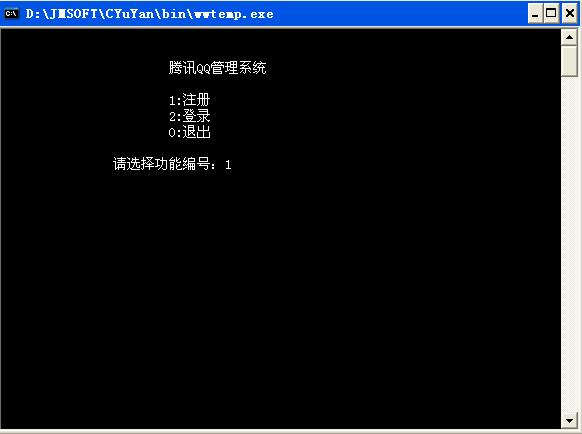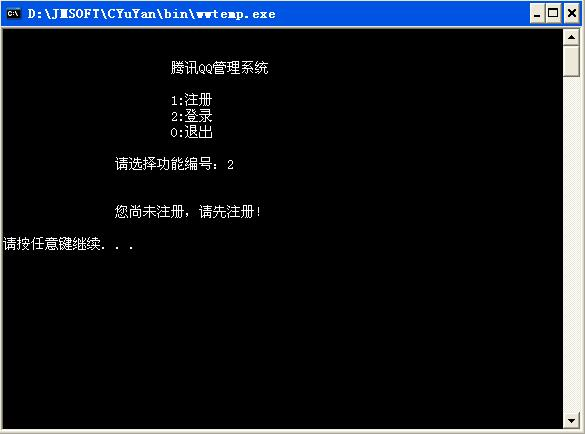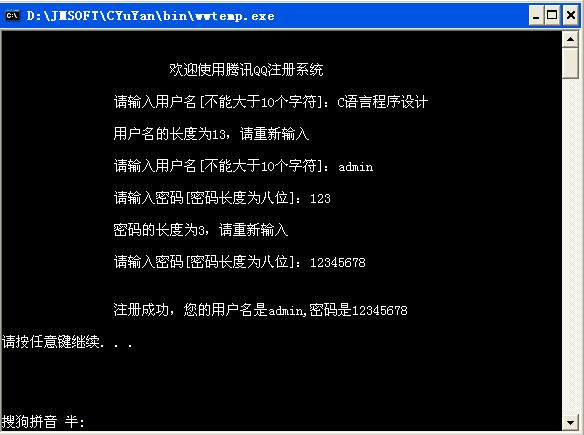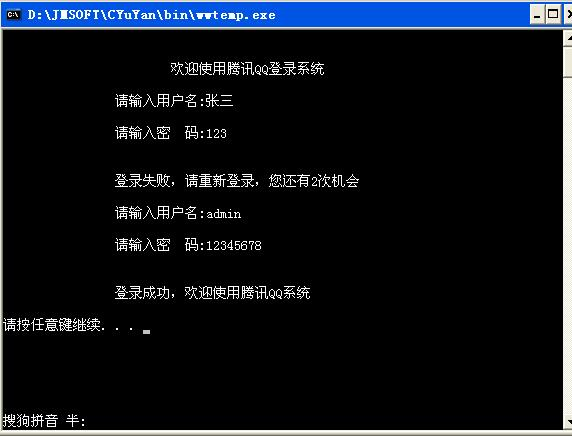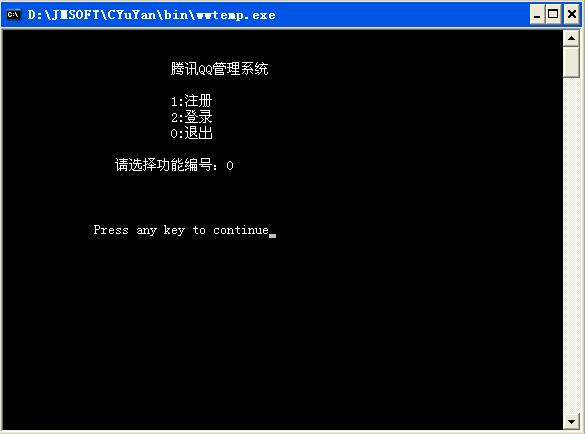展开全文• C语言内容，适合多个人群下载 基本的刚使用C语言的学生进行课程设计时可采用
• 本次课程设计使用C语言制作程序来实现图书的登记，删除，查询，浏览以及读者的借阅，还书，会员登录，修改密码等功能。程序中涉及到数据链表和文件指针的操作，包括结构体数据定义，使用及文件的读写，定位，修改等...
C课程设计——图书管理系统
1、题目意义
图书馆，作为文献的聚集地和展示平台，常常扮演着引领文化前进的角色，是每个大学不可或缺的基础设施，而图书管理系统则是一个图书馆能够正常运转的关键。本次课程设计使用C语言制作程序来实现图书的登记，删除，查询，浏览以及读者的借阅，还书，会员登录，修改密码等功能。程序中涉及到数据链表和文件指针的操作，包括结构体数据定义，使用及文件的读写，定位，修改等。
2、设计思想
①新建账号密码，然后通过二者的匹配登录系统，这样可以保证是本校同学才能使用，另外还可以修改密码。
②添加图书的基本信息，如 书号，书名，作者，出版社，类别，进库量；
③删除图书，通过图书的书名进行删除；
④图书查询，通过图书的书号，书名，作者或者类别进行检索；
⑤浏览图书的库存，将所有数目展示出来；
⑥借书登记，先判断是否有这本数，然后进行借书人学号，姓名，归还截止期限，借书书名进行登记
⑦还书情况查看。
⑧借阅情况查看。
⑨会员登录，通过数据文件中的已注册的账号密码来登录到图书管理系统。
⑩修改密码
3.总设计框架图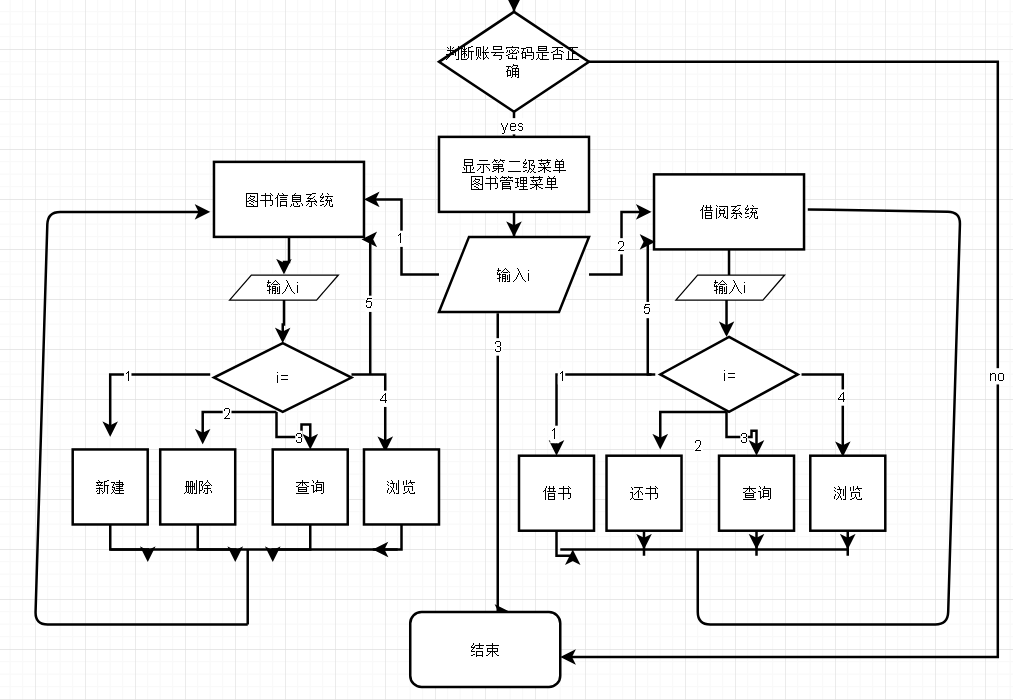4.代码展示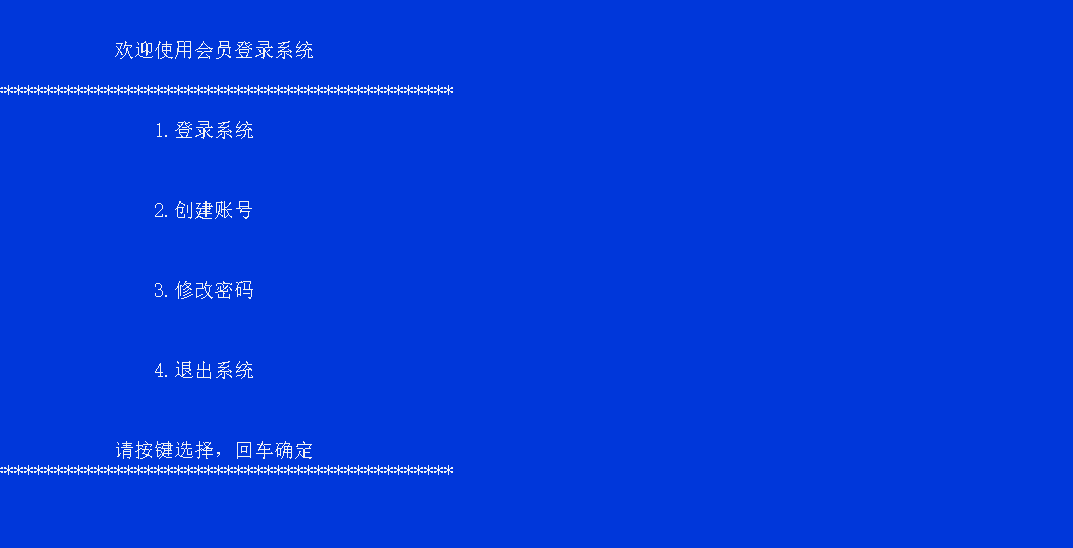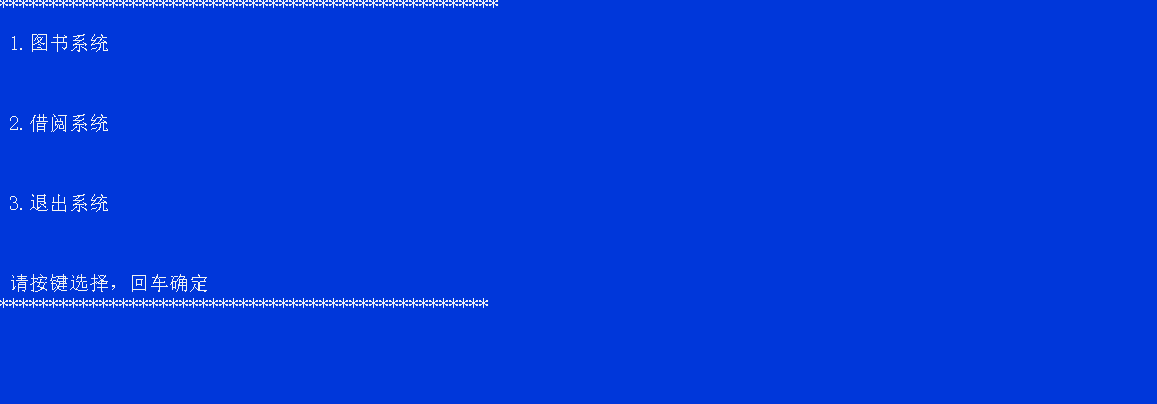#include <stdio.h>
#include <stdlib.h>//其中包含system函数
#include <conio.h>//定义了通过控制台进行 数据输入 和数据输出的函数，如getch函数。
#include <string.h>//定义字符数组
#include <math.h>
#define LEN sizeof(struct book)//有关图书信息的结构体
#define LEN2 sizeof(struct land)//有关登录系统的结构体

struct book//图书信息
{
char num,nam,aut,pub,cat;
int  many;
float price;
struct book*next;
};

{
int jnum;
char jnam,time,tsnam;
};
struct land//登录信息
{
int zhanghao;
struct land*next;
};

int tjzs();//统计library文本个数函数
void xg(int,char[] );

void main1();// 主菜单功能
void tsmain();//图书菜单功能
void cxts();//查询图书
void zjts();//增加图书
void scts();//删除图书
void llts();//浏览图书

void jmain();//读者借阅信息
void js();//借书
void hs();//还书
void cxjs();//查询借书
void lljs();// 浏览借书情况

void land();//登录功能系统
int xinjian(int,char[] );//创建账号密码
void xgmm();//修改密码
void lmain();//登录界面函数

int tjzs()//统计图书文本个数
{
FILE *fp;
int txcl=0,n;
float tprice=0;
char tname={'\0'},tauthor={'\0'},tchuban={'\0'},tkind={'\0'},tshuhao={'\0'};
fp=fopen("library.txt","r");//打开文件
for (n=0;!feof(fp);n++)//逐个读文件
fscanf(fp,"%s%s%s%s%s%d%f",tshuhao,tname,tauthor,tchuban,tkind,&txcl,&tprice);
n--;
fclose(fp);//关闭文件
return (n);//返回个数
}

int tjdzzs()//统计文读者本个数函数

{
FILE *fp;
int zhenghao=0,n;
char mingzi={'\0'},riqi={'\0'},zname={'\0'};
for (n=0;!feof(fp);n++)//逐个读文件
fscanf(fp,"%d%s%s%s ",&zhenghao,&mingzi,&riqi,&zname);
n--;
fclose(fp);//关闭文件
return (n);//返回个数
}

int tjzs3()//统计账号密码文本个数
{
FILE *fp;
int zhao=0,n;
char mm={'\0'};
fp=fopen("land.txt","r");//打开文件
for (n=0;!feof(fp);n++)//逐个读文件
fscanf(fp,"%d %s",&zhao,mm);
n--;
fclose(fp);//关闭文件
return (n);//返回个数
}

{
system ("cls");

printf("**************************************************");

printf("\n\n 1.图书系统\n\n");

printf("\n\n 2.借阅系统\n\n");

printf("\n\n 3.退出系统\n\n");

printf("\n\n 请按键选择，回车确定\n");

printf("*************************************************\n");

return ;
}

{
system ("cls");

printf("****************************************************");

printf("\n 1.增加图书\n\n");

printf("\n 2.删除图书\n\n");

printf("\n 3.查询图书\n\n");

printf("\n 4.库存浏览\n\n");

printf("\n 5.返回上一层\n\n");

printf("\n 请按键选择，回车确定\n");

printf("***************************************************\n");

return ;

}

void main1()
{
void tsmian();
void jmain();
char choose;
scanf(" %c",&choose);
switch(choose)//功能函数
{
case'1':
tsmain();
break;
case'2':
jmain();
break;
case'3':
system("cls");
getch();
exit(0);
system ("cls");
break;
}
}

void tsmain()
{
void zjts();
void scts();
void ctts();
void llts();
char choose;
scanf(" %c",&choose);
for(;;)
{
switch(choose)
{
case'1':
zjts();
break;
case'2':
scts();
break;
case'3':
cxts();
break;
case'4':
llts();
break;
case'5':
main1();
break;
}
}
}

void zjts()//增加图书
{
FILE*fp;
char i;
int many=0;
float price=0;
char  nam={'\0'},aut={'\0'},cat={'\0'},pub={'\0'},num={'\0'};
system ("cls");

if ((fp=fopen("library.txt","r"))==NULL)//if语句：打开图书馆文件，不存在此文件则新建
{
fp=fopen("library.txt","w");
fclose(fp);
}
fp=fopen("library.txt","a");

printf("\n请按以下格式输入图书信息:\n书号 书名 作者 出版社 类别 进库量 单价");

for(;i!=27;)//为了实现输入一次后按esc退出
{
printf("请输入:\n");
scanf("%s%s%s%s%s%d%f",num,nam,aut,pub,cat,&many,&price);
fprintf(fp,"%-8s%-9s%-14s%-16s%-18s%-7d%-8.2f\n",num,nam,aut,pub,cat,many,price);
printf("继续输入请按回车，结束输入请按Esc\n");
i=getch();//暂停程序当i接收后继续下一条指令
for (;i!=13&&i!=27;)//保证只能是CR和ESC才能退出循环,输入其他字符无用，暂停程序，按'CR'继续。
i=getch();
}

fclose(fp);
printf("\n保存成功，按任意键返回上一层!");
getch();
tsmain();//返回上一层
}

void scts()//删除图书
{
struct book *p,*p1,*p2;
int tmany=0,n=0,j,k;
float tprice=0;
char  tnam={'\0'},taut={'\0'},tcat={'\0'},tpub={'\0'},tnum={'\0'};
char jjnam={'\0'};
char i;
FILE *fp;
if ((fp=fopen("library.txt","r"))==NULL)//打开文件
{
system ("cls");
printf("\n记录文件不存在!按任意键返回");
getch();
tsmain();
}

else//实现删除的功能
{
system ("cls");
printf("\n请输入你要删除的书名:");//输入删除图书书名
scanf("%s",jjnam);
printf("\n确认删除请回车，取消请按Esc\n");
i=getch();
for(;i!=13&&i!=27;)
i=getch();
if (i==27)
tsmain();
fp=fopen("library.txt","r");
j=tjzs();

for (k=0;k<j;k++)
{
fscanf(fp,"%s%s%s%s%s%d%f",tnum,tnam,taut,tpub,tcat,&tmany,&tprice);
if (strcmp(jjnam,tnam))//比较名字，将不同名字的信息复制到链表
{
n++;//相同返回值为0不执行if语句继续循环，不同则执行直到将所有不同的书名建立成链表
if (n==1)//建立链表
{
p1=p2=(struct book*)malloc(LEN);
}
else
{
p2->next=p1;
p2=p1;
p1=(struct book*)malloc(LEN);//新建链表
}

strcpy(p1->num,tnum);//复制书号
strcpy(p1->nam,tnam);//复制书名
strcpy(p1->aut,taut);//复制作者名字
strcpy(p1->pub,tpub);//复制出版社
strcpy(p1->cat,tcat);//复制类别
p1->many=tmany;//复制个数
p1->price=tprice;//复制单价
}
}
if (n==0)//如果图书只有一项且这一项刚好和要删除的相同
{
}
else//建立链表的最后剩余一个储存空间，所以封底
{
p2->next=p1;
p1->next=NULL;
fclose(fp);
}
}

fp=fopen("library.txt","w");//清空文件，只写打开，然后关闭
fclose(fp);
fp=fopen("library.txt","a");//追加文件

for (;p!=NULL;)//把链表内容覆盖到文件
{
fprintf(fp,"%-8s%-9s%-14s%-16s%-18s%-7d%-8.2f\n",p->num,p->nam,p->aut,p->pub,p->cat,p->many,p->price);
p=p->next;
}
fclose(fp);
system ("cls");
printf("\n删除成功 \n按任意键返回上一层\n");
getch();//返回上一层
tsmain();
}

void cxts()//查询图书
{
FILE *fp;
int k=0,many=0,m=0,n=0;
float price=0;
char  nam={'\0'},aut={'\0'},cat={'\0'},pub={'\0'},num={'\0'};
char i;
char chazhao={'\0'};
if ((fp=fopen("library.txt","r"))==NULL)//打开文件
{
system ("cls");
printf("\n记录文件不存在!按任意键返回");
getch();
tsmain();
}
system("cls");
printf("请输入书号,书名,作者或类别查询：\n");
scanf("%s",chazhao);
system ("cls");
m=tjzs();

for (n=0;n<m;n++)
{
fscanf(fp,"%s%s%s%s%s%d%f",num,nam,aut,pub,cat,&many,&price);
if(!strcmp(chazhao,num)||!strcmp(chazhao,nam)||!strcmp(chazhao,aut)||!strcmp(chazhao,cat))
{
if(k==0)
{
printf("查询结果:\n\n");
printf("书号\t书名\t作者\t\t出版社\t\t类别\t\t现存量\t单价\n");
}
printf("%-8s%-9s%-14s%-16s%-18s%-7d%-8.2f\n",num,nam,aut,pub,cat,many,price);
k++;
}
}
if (k==0)//文件夹为空则输出无记录并返回上一层
{ system ("cls");
printf("\n无符合记录!\n");
getch();
tsmain();
}
fclose(fp);//查询结束
getch();
tsmain();
}

void llts()//浏览图书
{
FILE *fp;
int n=0;
int k=0,m=0,many=0;
float price=0;
char  nam={'\0'},aut={'\0'},cat={'\0'},pub={'\0'},num={'\0'};
char i;
if ((fp=fopen("library.txt","r"))==NULL)//打开文件
{
system ("cls");
printf("\n记录文件不存在!按任意键返回");
getch();//返回
tsmain();
}
n= tjzs();
if (n==0)
{
system ("cls");
printf("\n无任何记录!");
}

fp=fopen("library.txt","r");//打开只读文件
system ("cls");
printf("书号\t书名\t作者\t\t出版社\t\t类别\t\t库存量\t单价\n");

for (m=0;m<n;m++)//输出数据
{
fscanf(fp,"%s%s%s%s%s%d%f",num,nam,aut,pub,cat,&many,&price);
printf("%-8s%-9s%-14s%-16s%-18s%-7d%-8.2f\n",num,nam,aut,pub,cat,many,price);
}
fclose(fp);
printf("\n按任意键返回\n");
getch();
tsmain();
}

//~~~~~~~~~~~~~~~~~~~~~~~~~~~~~~~~~~~~~~~~~~~~~~~~~~~~~~~~~~~~~~~~~~~~~~~~~~~~~~~~~~~~~~~~~~~~~~~~~~~~~~~!~~~~~~~~~~~~~~~~~~~~~

{
system("cls");
printf("*****************************************************");
printf("\n\n 1.借书登记\n\n");
printf("\n\n 2.还书登记\n\n");
printf("\n\n 3.借阅情况查看\n\n");
printf("\n\n 4.查询借书\n\n");
printf("\n\n 5.返回上一层\n\n");
printf("\n\n 请按键选择，回车确定\n");
printf("****************************************************");
return ;
}

void jmain()//借阅系统函数
{
void js();
void hs();
void lljs();
void cxjs();
char choose;
scanf(" %c",&choose);
for(;;)
{
switch(choose)
{
case'1':
js();
break;
case'2':
hs();
break;
case'3':
lljs();
break;
case'4':
cxjs();
break;
case'5':
main1();
break;
}
}

}

void js()//借书函数
{
FILE *fp,*fp3;
struct book *p,*p1,*p2;
int i,loop,k,n=0,flag=0,s=0;
int jnum=0;
int many=0;
float tprice=0;
char tname={'\0'},tauthor={'\0'},tchuban={'\0'},tkind={'\0'},tshuhao={'\0'},
ttname={'\0'},mingzi={'\0'},riqi={'\0'},zname={'\0'};
char hit=0;

system("cls");
if((fp=fopen("library.txt","r"))==NULL)
{
system("cls");
printf("\n 图书馆无库存!按任意键退出!");
getch();
exit(0);
}
else
{
printf("\n请输入借阅书名:\n请输入:");
scanf("%s",zname);
k= tjzs();//统计图书馆文件个数

for (i=0;i<k;i++)//先将图书信息建立链表，更改库存
{
fscanf(fp,"%s%s%s%s%s%d%f",tshuhao,tname,tauthor,tchuban,tkind,&many,&tprice);
n++;
if(n==1)
{
p1=p2=(struct book*)malloc(LEN);
}
else
{
p2->next=p1;
p2=p1;
p1=(struct book*)malloc(LEN);

}
strcpy(p1->num,tshuhao);//复制书号
strcpy(p1->nam,tname);//复制书名
strcpy(p1->aut,tauthor);//复制作者
strcpy(p1->pub,tchuban);//复制出版社
strcpy(p1->cat,tkind);//复制类别
p1->many=many;//复制现存量
p1->price=tprice;//复制单价
}
if(n==0)
else
{
p2->next=p1;
p1->next=NULL;
fclose(fp);
}

}

for (;p!=NULL;)
{
if(!(strcmp(p->nam,zname)))//判断要借书的是否存在，标记等于1，存在库存减一
{

flag=1;
loop=p->many;
(p->many)--;
}
p=p->next;
}
if(flag&&(loop>0))//存在被借的图书且现存量大于0，把库存量变化后的链表存入文件
{

fp=fopen("library.txt","w");
fclose(fp);
fp=fopen("library.txt","a");

for(;p !=NULL;)
{
fprintf(fp,"%-8s%-9s%-14s%-16s%-18s%-7d%-8.2f\n",p->num,p->nam,p->aut,p->pub,p->cat,p->many,p->price);
p=p->next;
}
free(p);
fclose(fp);

{
fclose(fp3);
}

printf("\n请按以下格式输入读者信息:\n 证号 姓名 借书日期 借书书名\n请输入:");//录入读者信息
scanf("%d %s %s %s",&jnum,mingzi,riqi,zname);
fprintf(fp3,"\n%-8d%-23s%-18s%-10s\n",jnum,mingzi,riqi,zname);
fclose(fp3);
printf("借书成功!请两个月内归还！！！按任意键返回\n");
getch();//返回
jmain();//调用借阅系统
}
else
{
if(flag!=0)
printf("此书已被借完!按任意键返回!");//否则输出此书已被借完
else
printf("查找无此书!按任意键返回");
}
getch();//返回
jmain();//调用借阅系统

}

void hs ()//还书函数
{
FILE *fp,*fp3;
struct book *zp1,*lp1,*lp2;
int txcl=0,i;
float tprice=0;
char tname={'\0'},tauthor={'\0'},tkind={'\0'},
tchuban={'\0'},ttname={'\0'},tshuhao={'\0'};
int ttzhenghao=0,tzhenghao=0,n=0,k=0,t=0,flag=0;
char tmingzi={'\0'},triqi={'\0'},tzname={'\0'},ttzname={'\0'};
char hitkey=0;
system ("cls");
{
{
system ("cls");
printf("\n 不存在借书者!按任意键退出!");
getch();
system("cls");
jmain();
}

else
{{
printf("\n请输入读者证号和书名:\n请输入:");
scanf("%d %s",&ttzhenghao,ttzname);//输入还书证号和书名
k=tjdzzs();//获取读者文件夹信息个数
for (i=0;i<k;i++)//读取读者文件夹信息
{
fscanf(fp,"%d%s%s%s\n ",&tzhenghao,tmingzi,triqi,tzname);
if((ttzhenghao==tzhenghao)&&!strcmp(ttzname,tzname))//如果证号书名存在，则标记为1
flag=1;
}
fclose(fp);
if(flag)
{
for (i=0;i<k;i++)//将读者文件复制到链表
{
fscanf(fp,"%d%s%s%s\n ",&tzhenghao,tmingzi,triqi,tzname);//读取文件信息
if(!((ttzhenghao==tzhenghao)&&!strcmp(ttzname,tzname)))
{
n++;
if (n==1)
{
}
else
{
p2->next=p1;
p2=p1;
}
p1->jnum=tzhenghao;//复制证号
strcpy(p1->jnam,tmingzi);//复制读者名字
strcpy(p1->time,triqi);//复制日
strcpy(p1->tsnam,tzname);//复制书名
}}
if (n==0)
else
{
p2->next=p1;
p1->next=NULL;
fclose(fp);
}
fclose(fp);
for (;p!=NULL;)//把链表内容覆盖读者文件
{
fprintf(fp,"\n%-8d%-23s%-18s%-10s\n",p->jnum,p->jnam,p->time,p->tsnam);
p=p->next;
}
free(p);
fclose(fp);
}}}}

if(flag)//标记为1，即还书时更改库存
{{
{
printf("确认还书请按回车!");
for (;hitkey!=13&&hitkey!=27;)
hitkey=getch();
if (hitkey==13)
printf("成功!按任意键返回!");
n=0;flag=0;
fp3=fopen("library.txt","r");//打开图书馆文件
k=tjzs();//获取图书馆文件个数
for (i=0;i<k;i++)//将图书馆文件复制到链表
{
fscanf(fp3,"%s%s%s%s%s%d%f",tshuhao,tname,tauthor,tchuban,tkind,&txcl,&tprice);//读取信息
n++;
if (n==1)
{
lp1=lp2=(struct book*)malloc(LEN);//新建链表
}
else
{
lp2->next=lp1;
lp2=lp1;
lp1=(struct book*)malloc(LEN);//新建链表
}
strcpy(lp1->num,tshuhao);//复制书号
strcpy(lp1->nam,tname);//复制书名
strcpy(lp1->aut,tauthor);//复制作者
strcpy(lp1->pub,tchuban);//复制出版社
strcpy(lp1->cat,tkind);//复制类别
lp1->many=txcl; //复制现存量
lp1->price=tprice;//复制单价
}
if (n==0)
{
}
else
{
lp2->next=lp1;
lp1->next=NULL;
fclose(fp3);
}}}
for (;zp1!=NULL;)
{
if(!(strcmp(zp1->nam,ttzname)))//寻找书名相同
++(zp1->many);//现存量加1
zp1=zp1->next;
}
fp3=fopen("library.txt","w");//清空图书馆文件
fclose(fp);
fp3=fopen("library.txt","a");//追加信息
for (;zp1!=NULL;)//把链表内容覆盖图书馆文件
{
fprintf(fp3,"%-8s%-9s%-14s%-16s%-18s%-7d%-8.2f\n",
zp1->num,zp1->nam,zp1->aut,zp1->pub,zp1->cat,zp1->many,zp1->price);
zp1=zp1->next;
}
fclose(fp3);
getch();//返回
jmain();//调用借阅系统
}
else
printf("不存在此信息!按任意键返回!");
getch();//返回
jmain();//调用借阅系统
}

void lljs()//显示借书情况函数

{
FILE *fp;
int zhenghao=0,xcl=0,n=0,i=0,j=0;
char mingzi={'\0'},riqi={'\0'},zname={'\0'};
{
system ("cls");
printf("\n记录文件不存在!按任意键返回");
getch();
jmain();
}
n=tjdzzs();
if (n==0)
{ system ("cls");
printf("\n无任何记录!");
}
system ("cls");
printf("\n证号\t读者姓名\t\t借书日期\t书名\n");
for (;!feof(fp);)//输出文件信息
{
fscanf(fp,"%d%s%s%s\n ",&zhenghao,mingzi,riqi,zname);
printf("\n%-8d%-23s%-18s%-10s\n", zhenghao,mingzi,riqi,zname);
}
fclose(fp);
printf("\n按任意键返回\n");
getch();//返回
jmain();//调用借阅系统
}

void cxjs()//查询借书
{
FILE *fp;
int jsnum=0,k=0,many=0,m=0,n=0;

char  jsnam={'\0'},jstime={'\0'},tsnam={'\0'};
char i;
char chazhao={'\0'};
{
system ("cls");
printf("\n记录文件不存在!按任意键返回");
getch();
jmain();
}
system("cls");
printf("请输入证号或姓名查询：\n");
scanf("%s",chazhao);
system ("cls");
m=tjzs();
for (n=0;n<m;n++)
{
fscanf(fp,"%d%s%s%s",&jsnum,jsnam,jstime,tsnam);
if(!strcmp(chazhao,jsnam)||(('chazhao'-'0')==jsnum))
{
if(k==0)
{
printf("查询结果:\n\n");
printf("\n证号\t读者姓名\t\t借书日期\t书名\n");
}
printf("\n%-8d%-23s%-18s%-10s\n",jsnum,jsnam,jstime,tsnam);
k++;
}
}
if (k==0)//文件夹为空则输出无记录并返回上一层
{ system ("cls");
printf("\n无符合记录!\n");
getch();
jmain();
}
fclose(fp);//查询结束
getch();
jmain();
}

//~~~~~~~~~~~~~~~~~~~~~~~~~~~~~~~~~~~~~~~~~~~~~~~~~~~~~~~~~~~~~~~~~~~~~~~~~~~~~~~~~~~~~~~~~~~~~~~~~~~~~~~~~~~~~~~~~~~~~~~~~~~~~~~~~~~~~~

{

printf("\n\n\t    欢迎使用会员登录系统\n\n");
printf("**********************************************");
printf("\n\n\t\t1.登录系统\n\n");
printf("\n\n\t\t2.创建账号\n\n");
printf("\n\n\t\t3.修改密码\n\n");
printf("\n\n\t\t4.退出系统\n\n");
printf("\n\n\t    请按键选择，回车确定\n");
printf("**********************************************");
return ;
}

void lmain()//登录功能函数
{
void land();
void xinjian();
char choose;
scanf(" %c",&choose);
switch(choose)//功能函数
{
case'1':
land();
break;
case'2':
xinjian();
break;
case'3':
xgmm();
break;
case'4':
system("cls");
getch();
exit(0);
system ("cls");
break;
}
}

void xinjian()//新建账户密码
{
FILE *fp;
int zhanghao;
char hit=0;
if ((fp=fopen("land.txt","r"))==NULL)//if语句：打开图书馆文件，不存在此文件则新建
{
fp=fopen("land.txt","w");
fclose(fp);
}
system("cls");
fp=fopen("land.txt","a");
for(;;)//输入两次密码确认，两次相同才能确认
{
printf("\n请按以下格式输入账户:\n学号 密码\n");
printf("请输入:");
printf("再次输入密码:\n");
{
break;
}
else
{
printf("两次输入密码不一致，继续创建按回车，退出按ESC");
hit=getch();
if(hit=27)
system("cls");
lmain();
}
}
fclose(fp);
printf("创建成功，按任意键返回");
getch();
system("cls");
lmain();
}

int match(int m,char a)//匹配数据库中的账号密码
{
FILE*fp;
int n=0,i=0;
int zhanghao;

if ((fp=fopen("land.txt","r"))==NULL)//不存在读者文件
{
system ("cls");
printf("\n 还未存在用户!请新建账户");
getch();
system("cls");
lmain();

}

for(;!feof(fp);)
{
if(m==zhanghao)
{
return 1;
else
{
return -1;
}
}
}
return 0;
}

void land()//输入账户密码的登录函数
{
int zhanghao;
int i=2,j,k,n;
char hit=0;
system("cls");
do
{
printf("\n请输入账号:\n");
scanf("%d",&zhanghao);
printf("确认输入请安回车，重新输入请按ECS");
hit=getch();//暂停程序当i接收后继续下一条指令
for (;hit!=13&&hit!=27;)//保证只能是CR和ESC才能退出循环,输入其他字符无用，暂停程序，按'CR'继续。
{
hit=getch();
}
}
while(hit==27);
printf("\n请输入密码:\n");
if(i==1)
{
printf("登陆成功!按任意键继续");
getch();
main1();
}
else
{
if(i==-1)
{
printf("密码错误!");
getch();
land();
}
if(i==0)
printf("不存在此用户");
getch();
system("cls");
lmain();
}
}

void xg(int z,char m)//修改函数
{
FILE *fp;
int zhanghao1,n,j,k;
char mima1;

fp=fopen("land.txt","r");
j =tjzs3();

for (k=0;k<=j;k++)
{
fscanf(fp,"%d %s",&zhanghao1,mima1);
if (z!=zhanghao1)//比较名字，将不同名字的信息复制到链表
{
n++;//相同返回值为0不执行if语句继续循环，不同则执行直到将所有不同的书名建立成链表
if (n==1)//建立链表
{
p1=p2=(struct land*)malloc(LEN2);
}
else
{
p2->next=p1;
p2=p1;
p1=(struct land*)malloc(LEN2);//新建链表
}
p1->zhanghao=zhanghao1;
}
}
if (n==0)
{
}
else//建立链表的最后剩余一个储存空间，所以封底
{
p2->next=p1;
p1->next=NULL;
fclose(fp);
}
fp=fopen("land.txt","w");//清空文件，只写打开，然后关闭
fclose(fp);
fp=fopen("land.txt","a");//追加文件
for (;p!=NULL;)//把链表内容覆盖到文件
{
p=p->next;
}
fprintf(fp,"%d %s\n",z,m);
fclose(fp);
system ("cls");

}

void xgmm()//修改密码
{

FILE *fp;
int zh=0,k=0,many=0,m=0,n=0;
int chazhao,hit;
char  mm={'\0'};
char i;

if ((fp=fopen("land.txt","r"))==NULL)//打开文件
{
system ("cls");
printf("\n记录文件不存在!按任意键返回");
getch();
system("cls");
lmain();
}
system("cls");
printf("请输入你的帐号和旧密码：\n");
scanf("%d %s",&chazhao,mima);
m =tjzs3();
for (n=0;n<=m;n++)
{
fscanf(fp,"%d %s",&zh,mm);
if(zh==chazhao)
{
if(!strcmp(mm,mima))
{
printf("请输入新的密码");
scanf("%s",mima);
printf("再次输入密码:\n");
{
xg(chazhao,mima);
getch();
lmain();
system("cls");
}
else
{
printf("两次输入密码不一致,按任意键退出");
hit=getch();
system("cls");
lmain();

}
}
else
{
printf("旧密码错误，按任意键返回！");
getch();
system("cls");
lmain();

}

}
}
printf("不存在此账号，按任意键返回");
fclose(fp);//修改结束
getch();
system("cls");
lmain();
}

int main()
{
system("color 1F");
lmain();
return 0;
}


5.遇到的难点及解决办法
（1）不知道如何停止程序，以及只让程序在ESC和回车按下后才继续工作。
解：查阅资料getch()
所在头文件：conio.h
函数用途：从控制台读取一个字符，但不显示在屏幕上
函数原型：int getch(void)
返回值：读取的字符
代码： for(;i!=13&&i!=27;)
i=getch();
（2）不知道C语言未初始化的变量的值，导致无限循环。
解：全局变量 、静态变量初始值为0
局部变量，自动变量初始值随机分配
（3）不知道如何快速清理文件和新建文件
解：查阅览书本后知道，先只写文件，如果文件名和已存在的相同，那么就会先删除原有文件，而且如果不存在文件将会之间新建文件。
fp=fopen(“library.txt”,“w”); \清空
fclose(fp);
if ((fp=fopen(“library.txt”,“r”))==NULL)//快速新建
{
fp=fopen(“library.txt”,“w”);
fclose(fp);
}
（4）对a!=2和a=!2弄混淆
解：a!=2：表示a不等于“2”；
a=!2：表示a等于“!2”(0);
（5）编译正确，但是有的时候scanf语句好像没有执行，输入数据回车，程序没反应
解：查阅得知：
这种情况通常发生在前面已经有了输入语句，而当前的scanf是在接收字符(即用%c控制输入)时。由于前面的输入语句(不一定是scanf)把最后输入的’\n’遗留在了输入缓冲区，而当前的scanf("%c",…);又会把’\n’当一个字符接收，又由于scanf在%c控制下只接收一个字符，所以就不能接收正式输入的字符了。解决这一问题的最简单办法是在接收字符的scanf的控制符"%c"中的%前加一个空格写成" %c"，把前一次输入遗留在输入缓冲区里的所有广义空格(’ ‘、’\t’、’\n’等)都吸收掉。在接收字符的scanf前加getchar()等的办法其实是有漏洞的——当缓冲区里只遗留了一个广义字符时可正常工作，若多于一个则同样出错。 按回车没反应
（6），关于“feof”的误区
For(;!feof(fp)?;
n++;
n–;来判断文件里面字符的个数为什么错误，
查阅得知：
1.EOF
EOF是一个计算机术语，为End Of File的缩写，在操作系统中表示资料源无更多的资料可读取。资料源通常称为档案或串流。通常在文本的最后存在此字符表示资料结束。这个定义的意思是，文档的结尾都有一个隐藏字符”EOF”，当程序读取它的时候，就会知道文件已经到达结尾。通常使用while循环加EOF判断作为读取结束的标志。
EOF 的值通常为 -1，但它依系统有所不同。
2.feof()
feof()的原理：
feof()函数，并不是通过读取到文件的EOF来评判，这个文件是否为空。
对feof()来说,它的工作原理是，站在光标所在位置，向后看看还有没有字符。如果有，返回0；如果没有，返回非0。它并不会读取相关信息，只是查看光标后是否还有内容。
直接使用时的错误分析：
对于一个空文件来说，当程序打开它的时候，它的光标会停在文件的开头，但是由于文件里什么内容都没有存（但是EOF是存在的），即整个文件就存贮了一个EOF。当程序打开文件，并直接调用feof()时，这个函数就会站在光标的位置向后张望，结果就看见了EOF，然后就当然返回0了。所以使用feof（）函数时，必须要读取文件，让光标移动。
（7）错因：用正确的格式写入文件，结果却没有数据
解：没有及时fclose（fp）,不弥补数据储存的原理。
打开文件fopen函数把返回值赋给fp，用fclose关闭文件，如果不关闭文件就结束程序将会丢失数据，因为，在向文件里面写入数据时，是先将数据输入到缓冲区，待缓冲区从满后才正式输出给文件。因此用fclose函数关闭文件时，先把缓冲区中的数据输出到磁盘文件，然后才撤销文件信息区。

下载链接

链接：https://pan.baidu.com/s/13fFq7OwTb9ulWMRYHym-ZA 密码：4wbd

亲，点个赞
❤





展开全文项目
• ## C语言 编写登录系统

千次阅读 多人点赞 2016-09-27 21:02:58
可进行三次密码输入，若三次全部输入错误，则退出系统
#define _CRT_SECURE_NO_WARNINGS 1

#include "stdio.h"
#include"string.h"
int main()
{
int i = 0;
printf("请输入密码> ");
while (i<3 )
{
printf("\n");
{
printf("登陆成功\n");
break;
}
else
{
i++;
if (i != 3)
printf("密码输入错误，请重新输入> ");
}
}

if (i == 3)
printf("密码错误三次，程序退出\n");
system("pause");
return 0;
}
展开全文• #include "Head.h" #include "Chinese.c" #include "Mouse.c" #include "Login.c" #include "Exit.c" #include "Window.c" #include "OtIT.c" #include ... /* 登录*/ Exit(); /* 退出*/ } 。。。。。。。。。。。
#include "Head.h"#include "Chinese.c"#include "Mouse.c"#include "Login.c"#include "Exit.c"#include "Window.c"#include "OtIT.c"#include "Scanf.c"void initgr(void) /* BGI初始化 */{int  gd = DETECT, gm = 0;    /* 和gd = VGA,gm = VGAHI是同样效果 */registerbgidriver(EGAVGA_driver);/* 注册BGI驱动后没成绩不需求.BGI文档的支持运行 */initgraph(&gd, &gm, "");if(graphresult()){printf ("Graphics isn't available...");getch();exit(1);}MskindMath();/* 计算鼠标的样子*/Msinit();}void main(void){initgr();/* 初始化*/Login(); /* 登录*/Exit();  /* 退出*/}。。。。。。。。。。。
展开全文• ## C语言-用户登录系统

千次阅读 多人点赞 2020-01-10 20:25:53
"\t********* 用户登录系统 *********\n" ) ; printf ( "\t*******************************\n" ) ; printf ( "\n\n" ) ; printf ( "\tPlease enter username.\n" ) ; printf ( "\tusername:" ) ; ...linux
• Visual FoxPro 6.0开发的C语言题库系统。试卷系统通过自动组卷和人工组卷两种方式，包括后台数据库的建立、维护以及前端应用程序的开发两个方面。系统有安全的用户登录模块、快捷方便的浏览界面、操作方便的题库、...
•有问必答
• 高校水电费管理系统 C语言课程设计源文件 功能分为两大类 用户管理 费用管理 运用了结构体数组 指针 文件的保存 编译环境是v s 2010 可在主页文章中查看运行测试
• C语言做的银行系统，没有源代码，不过是一个不错的教程，可以看一下！
• C语言程序设计 教务系统管理 输入输出文件 成绩统计
• ## C语言实现登录注册

万次阅读 多人点赞 2019-01-27 09:36:52
可以用于c语言大作业的登录系统。下面分享一下具体的代码。 首先定义一个结构体来存放用户的注册信息，账号密码等。 typedef struct The_users //typedef 可以定义结构体别名 { char id; //账号 char pwd...
• ## C语言超市管理系统（c语言）

万次阅读 多人点赞 2020-07-01 12:29:10
超市管理系统 1.包括管理员和顾客 2.管理员有登入，录入商品信息，显示所有商品信息功能 3.顾客有购物车和结算功能 4.购物车具有显示商品列表，显示购物车商品，往购物车添加商品的功能 全部代码下载链接：点击前往...链表 算法
• C语言设计的交通管理系统，为期末的课程设计，包括交警登录和驾驶员登录，交警罚单的增加和删除等功能
• c语言 教务管理系统 用gcc编辑器编译 多个文件 使用c语言做的一个学生信息管理系统，里面有管理员和老师对学生信息的更改，使用双链表，存储在一个.dat文件中
• ## C语言图书管理系统

万次阅读 多人点赞 2017-06-20 10:06:53
学校的期大一末考试就是让设计个小项目，因为当时比较菜，感觉图书管理系统应该挺好写的，就选了这个。可能是最开始想的太简单了，在写的过程中还是遇到了各种各样的问题。最头疼的一次是我当我调用了保存文件的...
• 本项目是基于C语言开发的一个ATM机管理系统， 1、 用户信息包括：账户，用户姓名，性别，密码，存款金额，绑定手机号等。 2、 查余额：用户通过识别卡，输入密码，来查询余额。 3、 取钱：通过输入密码及取钱数量来...
• 2、学生登录，学生使用自己的账号密码登录系统，选择开启的门，即可开启门禁；此外系统还会记录下学生登录的信息，如登录账号、登录时间、开启哪间门；   3、管理员登录，管理员登录也是要输入特定的账号密码...
• 一个自己写的C语言图书管理系统程序，在此分享一下，一起学习
• C语言开发的彩票管理系统，分为用户注册登录模块验证模块，管理员发行彩票，查询用户信息，公证员开奖三个模块
• 函数名: settime功 能: 设置系统时间用 法: void settime(struct time *timep);程序例:#include #include int main(void){struct time t;gettime(&t);printf("The current minute is: %...
• 使用C语言对文件进行操作，实现管理员和员工的登录、员工添加、删除、修改、查询，以及员工签到的功能。
• ## C语言图书管理系统设计报告

万次阅读 多人点赞 2017-06-20 17:37:54
源代码：https://blog.csdn.net/k_young1997/article/details/73480766 XXXX大学 ...C语言课程设计报告 题 目 图书管理系统设计 专业班级 XXXX级计算机科学与技术本科X班 组 别 计科第...
• C语言考试管理系统 本项目分为学生端和管理端 学生端：注册、登录、答题、排名、发邮件、收邮件、题目的增删改查…… 管理端：查询修改用户、查询邮箱…… 管理端直接输入 账号：101 密码：54759 即可登录成功。
• ①新建账号密码，然后通过二者的匹配登录系统，这样可以保证是本校同学才能使用，另外还可以修改密码。 ②添加图书的基本信息，如 书号，书名，作者，出版社，类别，进库量； ③删除图书，通过图书的书名进行删除； ...
• 用纯C语言编写的简单的宿舍管理系统。包括文件的读写，信息的输入和输入；主要功能包括增删改、查看、检索等；输入输出在Dos界面。
• C语言系统登录中，密码加密函数中调用其他函数时，无法调用，而且导致现在不管输入什么 用户名和密码都能进入系统，求大佬解释，我认为是这个加密函数的问题，求大佬解释 ![图片说明]...c 加密...

# c语言登录系统如何做c语言 订阅